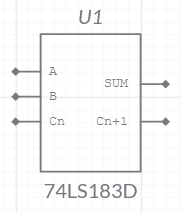OK

74LS183D is a carry-save full adder which features an individual carry output from each bit for use in multiple-input to produce the true sum and true carry outputsFunction table

Inputs Outputs
Cn
B A SUM Cn+1
L
L
L
L
L
L
L H
H
L
L H
L
H L
L H H
L
H
H
L
L
H L
H
L H L
H
H
H L L
H
H
H H
H
H

H = HIGH Level

L = LOW Level

X = Don't Care